# Effects of a moving mirror on a ray of light

• B
• yrjosmiel
In summary, in this conversation, the participants discuss the effects of a moving mirror on the path of a ray of light. They mention that the reflected dot will go up over time and that the angle of incidence and reflection may not match when the mirror is moving. They also consider the possibility of conducting an experiment with a spinning mirror to simulate the scenario. The conversation also touches upon the concept of length contraction and frames of reference. The participants conclude that the point where the light beam hits the wall will remain the same regardless of the frame of reference.f

#### yrjosmiel

Let's say I have an apparatus that's set up like this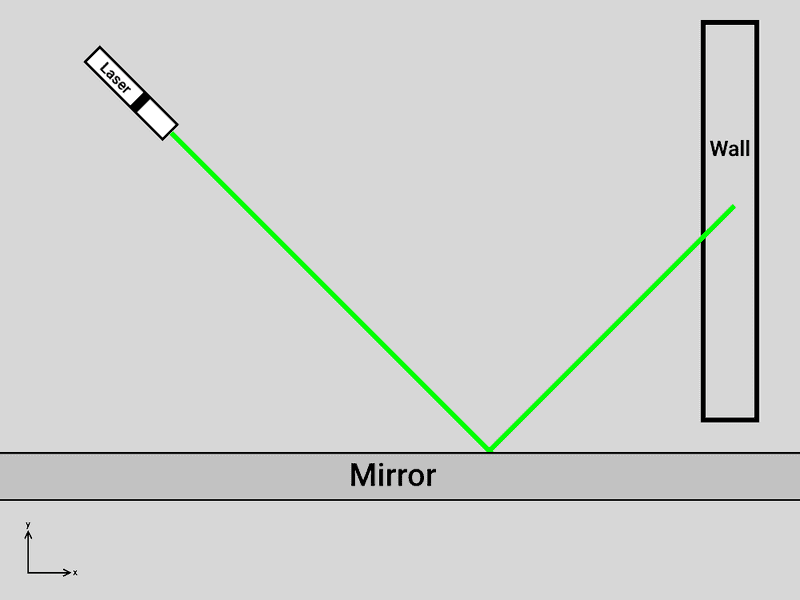Let's also say that the mirror is infinitely long.

If the mirror starts moving at subluminal speeds in the x axis, what would happen to the path of the ray of light? Would the dot made by the ray of light on the wall go up?

#### Attachments

Would the dot made by the ray of light on the wall go up?
The reflected dot will go up over time, yes.

I believe you will also find that the angle of incidence and the angle of reflection will not match. If you bounce the light from a stationary mirror and from a moving mirror that is [momentarily] at the same position, the reflected light from the moving mirror will strike the screen farther up. But I do not have any calculations to back that up with.

The reflected dot will go up over time, yes.
That's if the mirror is accelerating to subluminal speeds, right?
If the mirror was moving at subluminal speeds from the very beginning, it will stay fixed, but at least still higher than when the mirror is stagnant, right?

Has anyone done an experiment on this? Would it be worth doing an experiment on? Of course, we don't have an infinitely long mirror at our disposal but I think a spinning mirror can emulate the scenario.

Not certain what's being asked, however, since momentum is transferred to the light beam, it will be doppler shifted upon reflection.

That's if the mirror is accelerating to subluminal speeds, right?
No. That's if the mirror is moving at all. Try it with your bathroom mirror. Move a flashlight toward it and watch the reflected spot.

The reflected dot will go up over time, yes.
I thought this meant that the reflected dot will gradually go up.

I thought this meant that the reflected dot will gradually go up.
Yes, the reflected dot will gradually go up as the mirror goes up. What's the confusion?

If the mirror starts moving at subluminal speeds in the x axis,
Oh... on the _x_ axis. Never mind. No effect.

•yrjosmiel
No effect.
Hmmm. I was expecting for the reflected dot to actually go up due to special relativity. Length contraction was what I had in mind.
Let's say that the angle of reflection is θ. In this case, let's also say θ = 45° (this is with respect to the line perpendicular to the surface of the mirror). When the mirror is moving at subluminal speeds, it may seem to have no effect at first. However, when I shift the reference from the the laser to the mirror, the laser is now the one in motion. I thought that since the laser is the one moving in the mirror's reference frame, the laser would appear to be contracted. When you shorten one of the legs of a right triangle, the angles change. In turn, the angle of reflection will change to something less then 45°.

If the mirror starts moving at subluminal speeds in the x axis, what would happen to the path of the ray of light? Would the dot made by the ray of light on the wall go up?
We have two frames: one in which the mirror is moving while the wall and light source are at rest; and one in which the mirror is at rest while the wall and light source are moving. The angle that the light makes at the mirror is different in the two frames, but the point where the light beam hits the wall is the same.

•yrjosmiel
We have two frames: one in which the mirror is moving while the wall and light source are at rest; and one in which the mirror is at rest while the wall and light source are moving. The angle that the light makes at the mirror is different in the two frames, but the point where the light beam hits the wall is the same.
The point is still the same because the distance between the light source and the wall changed too, right?

Tennis players and table tennis players have hit techniques named slice, cut or top spin with moving their rackets during hit and controlling hit ball's moving direction. Don't moving mirrors play similar role with rackets for light?

We have two frames: one in which the mirror is moving while the wall and light source are at rest; and one in which the mirror is at rest while the wall and light source are moving.

Let us name these as Frame 1 and Frame 2, where mirror is moving relative to laser and wall.
In addition to these let us name frames in original physical condition, where all are at relatively rest.
Frame 3 : one in which the mirror, the wall and light source are all at rest
Frame 4 : one in which the mirror, the wall and light source are all moving with the same speed

Hit points are same in Frame 1 and Frame 2
Hit points are same in Frame 3 and Frame 4

But we have no reason to think that hit point 1,2 and that of 3,4 are same.

Last edited:
A complete analysis is fairly straightforward, although the algebra's a mess at the end. You can easily write down the path followed by a pulse of light emitted by the laser in the laser's frame such that it strikes the mirror at x=y=t=0. You can transform that to the mirror frame and calculate the reflected path - since the mirror's at rest, the law of reflection is trivial. Then you can transform back. The result I came up with is that I agree with the consensus - no effect.

•Dale
If there was an effect it would be seen in everyday observations involving reflections.

If there was an effect it would be seen in everyday observations involving reflections.
I don't recall mirrors moving at subluminal speeds being part of my "everyday observations".

•phinds
Isn't this what the Earth does when it moves in its orbit.
Stellar aberration.
Put a telescope on the mirror surface and the laser angle from the horizontal will decrease as the laser location will have shifted in the direction of motion.
The reflected ray will have its angle shifted down also.
The point of contact with the wall should appear to have become lower. YES?

Google finds http://dx.doi.org/10.1119/1.4720389, "A general principle for light reflecting from a uniformly moving mirror: A relativistic treatment", Galli & Amir.

Abstract said:
We investigate the reflection of light from a mirror moving at relativistic speeds and introduce a general principle to determine the relationship between the incident and reflected angles. This principle states that the momentum change of each photon is perpendicular to the surface of the mirror. We carry out sample calculations for various geometries.

I'd suggest trying their treatment, if one can get past the paywall, also analyzing it in the mirror frame, where we know the angle of incidence should be equal to the angle of reflection. I haven't attempted either at this point, though.

This paper cites some other papers on the same topic, so it'd probably be a good idea to read those, too.

We have to put mass of mirror is infinite so that we can neglect repulsion of mirror even in case light becomes high energy gamma ray in moving mirror IFR.

If the mirror starts moving at subluminal speeds in the x axis, what would happen to the path of the ray of light? Would the dot made by the ray of light on the wall go up?
If it's an ideal mirror, which exchanges momentum along the y-axis only, then there will be no effect from it's movement along x. It's analogus to collision with an ideal friction-less surface.

If it's an ideal mirror, which exchanges momentum along the y-axis only, then there will be no effect from it's movement along x. It's analogus to collision with an ideal friction-less surface.
Indeed.

In fact, you can use the y-axis-only property of the reflection to follow my earlier procedure without doing any maths. The four momentum of the incoming light in the laser/wall frame is ##(p_t,p_x,p_y,0)##, which becomes ##(p_{t'},p_{x'},p_y,0)## in the mirror frame, since ##p_{y'}=p_y##. The reflection just flips the sign on the y' component, and the inverse transform takes the momentum back to ##(p_t,p_x,-p_y,0)##. So there's no effect.

•TSny
I don't recall mirrors moving at subluminal speeds being part of my "everyday observations".

A subluminal speed is any speed less than the speed of light. I'm guessing that you meant to refer to "relativistic speeds", in other words speeds close to the speed of light.

If it's an ideal mirror, which exchanges momentum along the y-axis only, then there will be no effect from it's movement along x. It's analogus to collision with an ideal friction-less surface.

That's my analysis as well, based on analyzing the reflection from a stationary mirror in a moving frame. We can model the light be either its 4-frequency, or it's energy momentum 4-vector. The y-momentum of the light pulses won't be changed by the boost, the x-momen will be changed in identical ways for the downwards and upwards pulse. The result is that the angle of reflection should be equal to the angle of incidence in the moving frame as well as in the stationary frame.

At least according to my analysis, I've quoted the references google found but not read them due to a lack of access.

Not certain what's being asked, however, since momentum is transferred to the light beam, it will be doppler shifted upon reflection.

Finally, I understand what's being asked. No momentum is transferred to the beam in the rest frame of wall and laser. The boundary conditions on the surface of the mirror are unchanged by the translation speed IMO.

It would seem that the reflective properties of a real mirror are frequency or wavelength dependent. For example in the x-ray bands most household mirrors will become more transparent. If the mirror is normal to the beam and moving toward the laser fast enough, the beam in the mirror rest frame would blue shift to x-rays and the mirror start to become transparent. As the mirror normal is rotated  away from the beam direction the fresnel coefficients in the rest frame of the mirror are determined by the material properties in x-rays. Near grazing to the mirror surface the reflectivity will increase. This increase in near grazing incidence is how x-ray telescope optics are made. For the original post, the question is at what angle is the laser beam hitting the mirror in the mirrors rest frame?

 both beam and velocity vectors left unchanged as the mirror normal rotates.

For the original post, the question is at what angle is the laser beam hitting the mirror in the mirrors rest frame?
I've done some solving and I'm not sure if I'm right, but here's what I got anyway: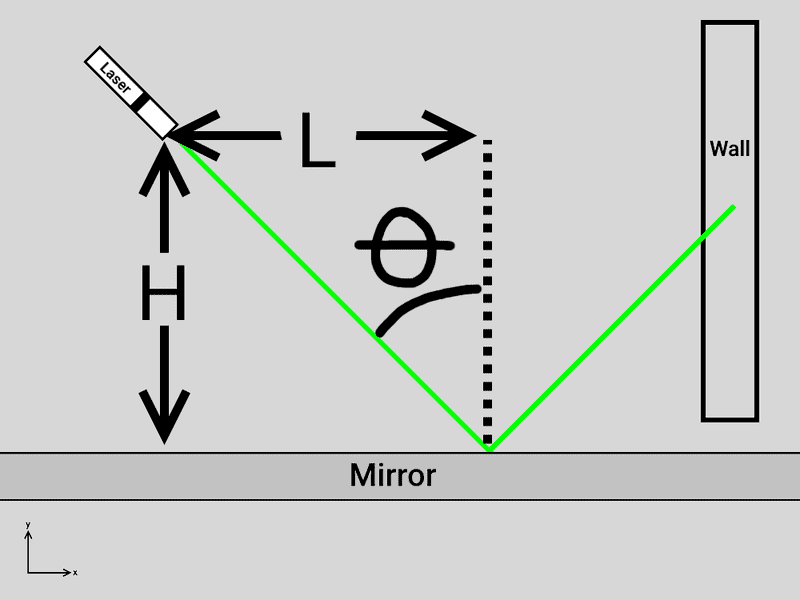let θo be the original angle of reflection (with respect to the perpendicular of the surface of the mirror)
θf be the final angle of reflection
Lo be the original distance (just in the x axis) from the light source to the point where the light ray hits the mirror
Lf be the final distance

If I recall my high school trigonometry,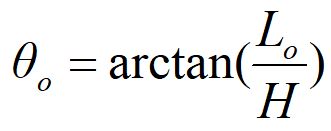Once the mirror starts moving, the distance L will appear to be shorter in the mirror's reference frame and will contract to Lf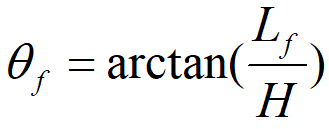How much a length is contracted is determined by: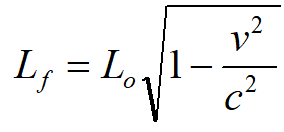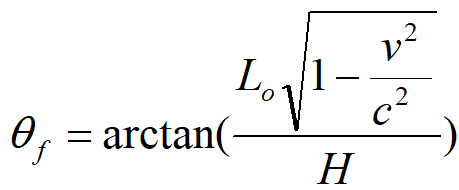#### Attachments

•Paul Colby
I've done some solving and I'm not sure if I'm right, but here's what I got anyway:
View attachment 226377
let θo be the original angle of reflection (with respect to the perpendicular of the surface of the mirror)
θf be the final angle of reflection
Lo be the original distance (just in the x axis) from the light source to the point where the light ray hits the mirror
Lf be the final distance
What is "original" vs. "final"? Before vs. after reflection? Stationary vs. moving mirror?

What is "original" vs. "final"? Before vs. after reflection? Stationary vs. moving mirror?
Sorry: the "original" is when the mirror is not moving. The "final" is when the mirror is moving.

Sorry: the "original" is when the mirror is not moving. The "final" is when the mirror is moving.
Okay, so what is your conclusion or remaining question (if any)?

Okay, so what is your conclusion or remaining question (if any)?
So far, after taking into account the contraction of the distance of the light source and the wall, I concluded that there is indeed no difference in where the laser dot will land on the wall.

If I do have any remaining questions: should I do an experiment to confirm this conclusion or would that be a waste of time and resources?

should I do an experiment to confirm this conclusion

How fast do you think you need to move the mirror to see an effect if you are wrong?

So far, after taking into account the contraction of the distance of the light source and the wall, I concluded that there is indeed no difference in where the laser dot will land on the wall.

If I do have any remaining questions: should I do an experiment to confirm this conclusion or would that be a waste of time and resources?
This open-access paper presents some relevant considerations:
Classical dynamics of a moving mirror due to radiation pressure

reflection rule is in approxation that mirror is massive enough. Compton effect should be active for high energy photon and mirror.

My understanding that the speed of light is slower in a medium is not because the light actually travels at a slower speed, but because the light interacts with the various particles of the medium which takes time. doesn't the mirror absorb the light than readmit it? wouldn't the mirror have at least traveled a short distance during this time? Wouldn't this cause the dot to be slightly lower?

My understanding that the speed of light is slower in a medium is not because the light actually travels at a slower speed, but because the light interacts with the various particles of the medium which takes time. doesn't the mirror absorb the light than readmit it? wouldn't the mirror have at least traveled a short distance during this time? Wouldn't this cause the dot to be slightly lower?
The initial question and all the answers have been assuming an ideal mirror, one that is of zero thickness and reflects 100% of the incident light.